Name:    3rd Grade Math Proficient Test 5

Multiple Choice
Identify the choice that best completes the statement or answers the question.

1.

Geometry – Congruency & Similarity – RIT 201 – 210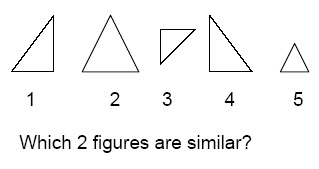a. 1, 4 d. 1, 2 b. 2, 4 e. 1, 3 c. 3, 5

2.

Geometry – Properties and Terminology- RIT 201 – 210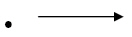a. a ray d. an acute angle b. an angle e. a line segment c. a line

3.

Which figure shows the circle’s diameter?
 a.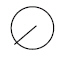d.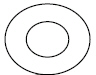b.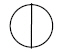e.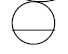c.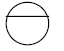4.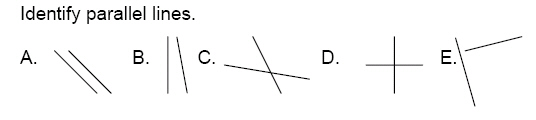a. A d. D b. B e. E c. C

5.

Geometry – Shapes and Figures – RIT 201 – 210
How many edges on a square pyramid?a. 4 d. 7 b. 6 e. 8 c. 5

6.

Which polygon is a pentagon?
 a.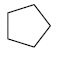d.b.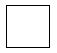e.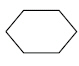c.7.

Which figure is not a quadrilateral?
 a.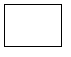d.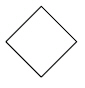b.e.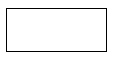c.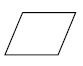8.

You can make a cylinder with:
 a. 3 circles d. 1 rectangle and 2 squares b. 1 circle and 1 cone e. 2 circles c. 2 circles and 1 rectangle

9.

Geometry – Symmetry and Transformations – RIT 201 – 210
Which figures are mirror images of each other?
 a.b.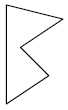c.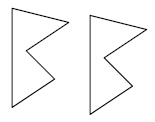d.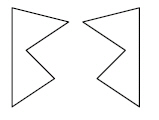e.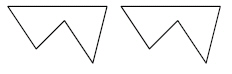10.

Geometry – Congruency and Similarity – RIT 211 – 220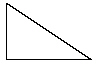Which figure is similar to the given figure?
 a.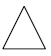d.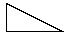b.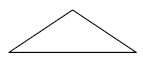e.c.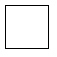11.

Triangle ABC is congruent to triangle RST.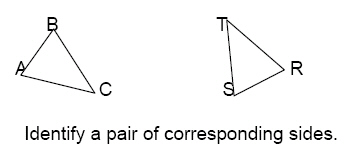a. AB and TR d. TR and TS b. BC and ST e. AB and TS c. AB and BC

12.

Geometry – Properties and Terminology – RIT 211 – 220a.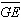d.b.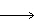CF e.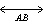c.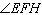13.a. A and B d. D and B b. A and C e. A and D c. B and C

14.

Triangle ABC is similar to Triangle LMN.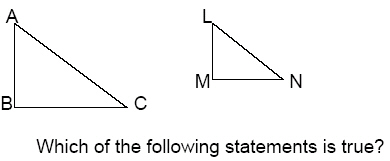a.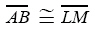d.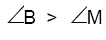b.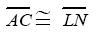e.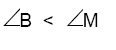c.15.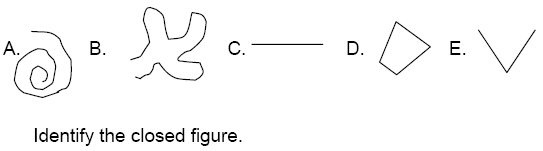a. A d. D b. B e. E c. C

16.

What is the name of a polygon with six sides?
 a. triangle d. hexagon b. quadrilateral e. octagon c. pentagon

17.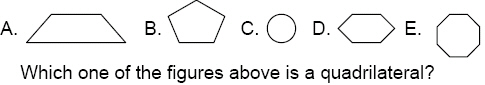a. A d. D b. B e. E c. C

18.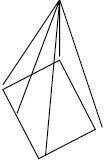How many vertices does a rectangular pyramid have?
 a. 4 d. 7 b. 5 e. 8 c. 6

19.

Geometry – Symmetry and Transformations – RIT 211 – 220

Identify the type of transformation.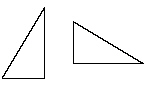a. translation d. symmetry b. rotation e. congruent c. reflection

20.

Measurement – Area, Perimeter, Circumference – RIT 191 – 200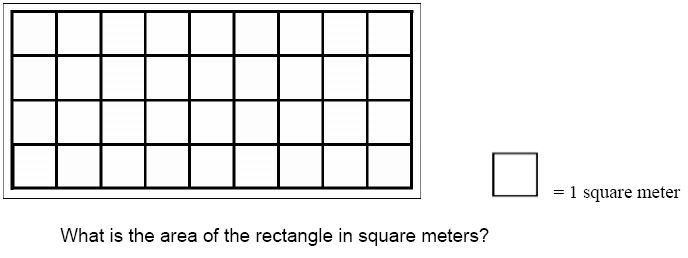a. 9 d. 13 b. 32 e. 50 c. 36

21.a. 12 d. 30 b. 10 e. 16 c. 14

22.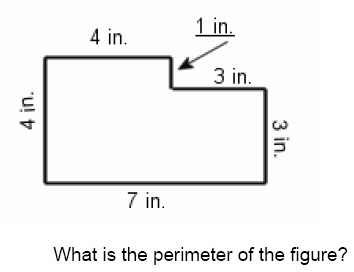a. 11 inches d. 21 inches b. 16 inches e. 22 inches c. 14 inches